# Computer Science : Algorithm Analysis

## Example Questions

← Previous 1

### Example Question #25 : Program Analysis

public void countStatements() {

       int a = 10, b = 15, c = 7;

       // Start

       for(int i = 0; i < a; i += 2) {

              b--;

              System.out.print("*");

       }

       for(int i = b; i >=0; i--) {

              for(int j = 0; j < c; j++) {

                     System.out.print("*");

              }

       }

       // End

}

In the code above, how many times will System.out.print be called between the comments "// Start" and "// End"?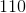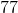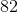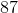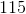Explanation:

Start by counting the number of times the first loop will cycle. Notice that the loop control variable (namely, i) is increased by 2 for each looping. This means that you will loop for i = 0, 2, 4, 6, 8. It will not loop for 10, for then, i will equal a. That will terminate the loop. Thus, you have five loopings thus far. Note, also that this means that b is decremented 5 times. Thus, at the end of this loop, b now equals 10.

For the second loop, you must be careful, for it has two loops. The outer loop will run from i = 10 to i = 0.  This is a total of 11 loopings. (This is important to note because it runs not only for 10 through 1 but also for the final number, 0. The loop within that loop will run from j = 0 to j = 6 (i.e. one less than c, which is 7). This is a total of 7 loopings. Therefore, these nested loops will run a total of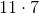or 77 times.  The total calls to System.out.print will be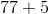, or 82.

### Example Question #1 : Counting Statement Executions

public void countStatements() {

       int[] array = new int;

       for(int i = 0; i < array.length; i++) {

              array[i] = 3 * i + 1;

              System.out.println("Yay!");

       }

       for(int i = 0; i < array; i++) {

              array[i]--;

              System.out.println("Yay!");

       }

       for(int i = array; i > 0; i--) {

              System.out.println("Yay!");

       }

}

How many calls will be made to System.out.println in the code above?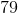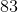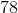Explanation:

Let us count for each loop, paying attention to variables as needed. The first loop will run a total of 54 times. Thus it will produce 54 total executions. Notice also how it initializes the array. This will be used in the second loop. Each element is equal to 3i + 1. Thus, we will have:

a = 1; a = 4; a = 7; a = 10; a = 13; a = 16; etc.

Now, loop two will use a in controlling its execution. Notice that it runs from i = 0 to i = 15. For each execution, it reduces the value of a[i] by one. Thus, be careful. It will eventually run for a, making it to be 15. This will then be used for the loop control. This means that it actually runs only from i = 0 to i = 14. Thus, the loop runs a total of 15 times.

The last loop runs from i = 9 to i = 1. (Note: This is because of the decrement in the second loop.) This is a total of 9 executions.

Thus, the total count of executions is: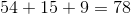### Example Question #27 : Program Analysis

How many statements are executed in the code snippet below?

for (int i = 0; i < arr.length; i++) {

if (i == 2) {

i++;

}

}

6

2

3

5

5

Explanation:

The statements executed in the code snippet are in order:

1. int i = 0
2. i < arr.length
3. i++ (which is equivalent to i = i + 1)
4. i == 2
5. i++

These statements are all executions that happen in order each time the for loop is run. Sometimes there may only be 4 executions if the if statement is false.

### Example Question #28 : Program Analysis

Consider the following code: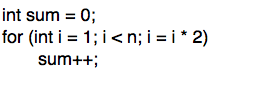What is the run-time of the code (in Big-O notation?)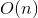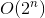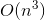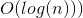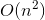Explanation:

The run-time can best be analyzed by breaking the code down by line. The line iterating the value of sum is performed in constant time, or O(1). The "for" loop that controls this constant-time operation is initialized at i = 1, and i is iterated by multiplying by 2 until it is equal to or greater than n. Thus, this loop will run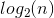times, and the run-time will be O(log(n))

### Example Question #29 : Program Analysis

Consider the following code: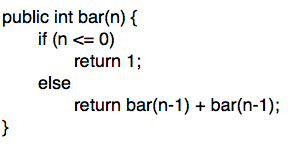What is the run time of the code (in Big-O notation?)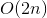Explanation:

The run time of this code is best analyzed by plugging in numbers. Take the value of bar(2) for example. bar(2) leads to two calls to bar(1), which lead to four calls to bar(0). A call to bar(4) leads to two calls to bar(3), four calls to bar(2), eight calls to bar(1), and sixteen calls to bar(0). Thus, it can be shown that the number of calls is proportional to 2^n, and that that run time is O(2^n).

### Example Question #30 : Program Analysis

Consider the following code: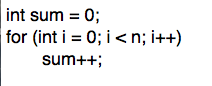What is the expected runtime of the code (in Big-O notation?)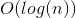Explanation:

The run-time can best be analyzed by breaking the code down by line. The line iterating the value of sum is performed in constant time, or O(1). This constant time operation is performed n times in the for loop, leading to a run time that is proportional to n, or O(n).

### Example Question #1 : Algorithm Analysis

Consider the following code: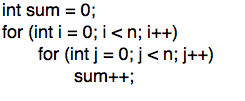What is the expected run time of the code (in Big-O notation?)Explanation:

The run time can best be analyzed by breaking the code down by line. The line iterating the value of sum is performed in constant time, or O(1). This constant time operation is performed n times in the inner "for" loop, and n times in the outer "for" loop. Thus, the run time is.

### Example Question #2 : Algorithm Analysis

Consider the following code: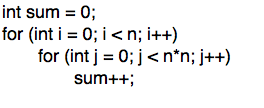What is the run time of the code (in Big-O notation)?Explanation:

The run time can best be analyzed by breaking the code down by line. The line iterating the value of sum is performed in constant time, or O(1). This constant time operation is performed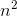times in the inner "for" loop, andtimes in the outer "for" loop. Thus, the overall run time is.

### Example Question #3 : Algorithm Analysis

What is the BigO of the following function?

public void bigO(int[][] m)
{
if (m.length > 2)
{
for (int i = 0; i < m.length - 1; i++)
{
for (int j = 0; j < m[i].length; j++)
{
System.out.println(m[i][j]);
}
}
}
else
{
for (int j = 0; j < m.length; j++)
{
System.out.println(m[j]);
}
}
}

O(1)

O(n)

O(log(n))

O(n*log(n)

O(n2)

O(n2)

Explanation:

The function is O(n2) because it has a nested loop of size 2, with no extras thrown in that could possibly add on a log(n). A good rule of thumb is this: if there are nested loops, then the BigO is usually O(nm), with m being the levels of loops in the longest part.

### Example Question #4 : Algorithm Analysis

Which is more efficient (i.e. Lower Big O)?

1)

arr = [1, 2, 3, 4, 5, 6, 7, 8]

arr2 = [[1,2],[3,4],[5,6], [7,8], [9,10], [10, 11]]

for (int i = 0; i < arr.length; i++) {

for (int j = i; j < arr2.length; j++) {

arr[i][j] = 0;

}

}

2)

arr = [1, 2, 3, 4, 5, 6, 7, 8]

arr2 = [[1,2],[3,4],[5,6], [7,8], [9,10], [10, 11]]

for (int i = 0; i < arr.length; i++) {

for (int j = 0; j < arr2.length; j++) {

arr[j] = 0;

}

}

The Big O is equivalent

Irrelevant

1

2